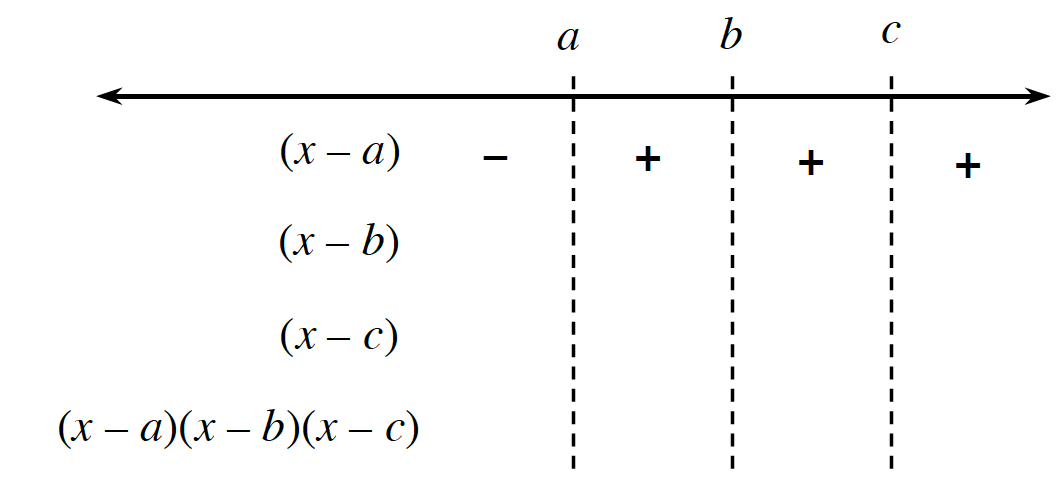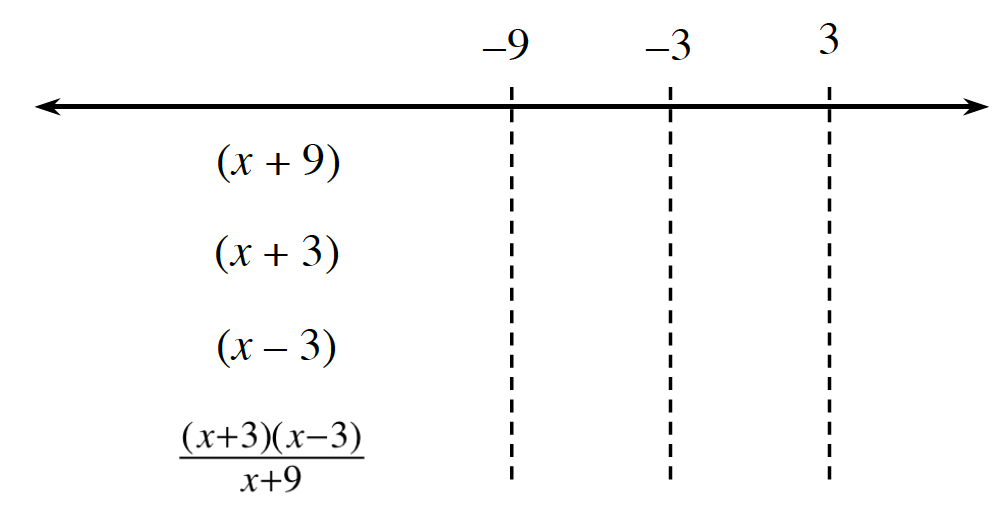### Home > PC3 > Chapter 7 > Lesson 7.1.5 > Problem7-67

7-67.

Solve each of the following inequalities.

1. $x^3+54>$$-2x^2+57x$

$x^3+2x^2-57x+54>0$

The possible roots of the polynomial are factors of $54$. Use polynomial division to determine the roots.

$(x-a)(x-b)(x-c)>0$

Where $a$, $b$, and $c$ are the roots of the polynomial.

Create and complete a sign chart like the one below to determine the intervals over which the polynomial is positive.1. $\frac { x ^ { 2 } + x } { x + 9 } \leq 1$

$\frac{x^2+x}{x+9}-1\le 0$

$\frac{x^2+x-(x+9)}{x+9}\le 0$

$\frac{(x+3)(x-3)}{x+9}\le 0$

Create and complete a sign chart like the one below to determine the intervals over which the polynomial is negative or $0$. Remember that $x=-9$ must be excluded from the solution.